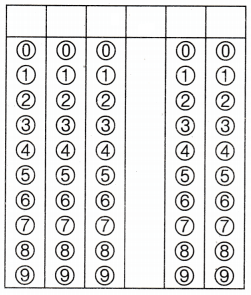Refer to our Texas Go Math Grade 4 Answer Key Pdf to score good marks in the exams. Test yourself by practicing the problems from Texas Go Math Grade 4 Module 7 Assessment Answer Key.

Vocabulary

• Distributive Property
• factor
• partial products

Choose the best term from the box to complete the sentence.

Question 1.
To find the product of a two-digit number and a 1-digit number, you can multiply the tens, multiply the ones, and find the sum of each ______________ .
To find the product of a two-digit number and a 1-digit number, you can multiply the tens, multiply the ones, and find the sum of each factor.

Question 2.
The _____________ states that multiplying a sum by a number is the same as multiplying each addend by the number and then adding the products.
The Distributive Property states that multiplying a sum by a number is the same as multiplying each addend by the number and then adding the products.

Concepts and Skills

Find the product.

Question 3.
6 × 10 = ___________
6 × 10 = 60.

Explanation:
The product of 6 × 10 is 60.

Question 4.
7 × 100 = ___________
7 × 100 = 700.

Explanation:
The product of 7 × 100 is 700.

Question 5.
9 × 300 = ___________
9 × 300 = 2,700.

Explanation:
The product of 9 × 300 is 2,700.

Question 6.
8 × 7,000 = ___________
8 × 7,000 = 56,000.

Explanation:
The product of 8 × 7,000 is 56,000.

Use grid paper or base-ten blocks to model the product. Then record the product.

Question 7.
6 × 15 = ___________
6 × 15 = 90.

Explanation:
Given that to construct a block of 6 × 15 which is 90.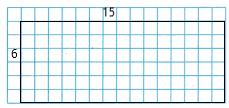Question 8.
3 × 18 = ___________
3 × 18 = 54.

Explanation:
Given that to construct a block of 3 × 18 which is 54.Question 9.
9 × 15 = ___________
9 × 15 = 135.

Explanation:
The product of 9 × 15 is 135.

Question 10.
8 × 17 = ___________
8 × 17 = 136.

Explanation:
The product of 8 × 17 is 136.

Record the product. Use expanded form to help.

Question 11.
5 × 64 = ___________
5 × 64 = 320.

Explanation:
The expanded form of 5 × 64 is
5 × 64 = 5 × (60+4)
= (5 × 60) +(5 × 4)
= 300+20
= 320.

Question 12.
3 × 272 = ___________
3 × 272 = 1,116.

Explanation:
The expanded form of 3 × 272 is
3 × 272 = 3 ×(300+70+2)
= (3 × 300)+(3 × 70)+(3 × 2)
= 900+210+6
= 1,116.

Estimate. Then record the product.

Question 13.
Estimate: __________Estimated product is 400,
Actual product is 375.

Explanation:
The product of 75×5 is 375 and the estimated product is 80×5 which is 400.Question 14.
Estimate: __________Estimated product is 3,900,
Actual product is 3,882.

Explanation:
The product of 647×6 is 3,882 and the estimated product is 650×6 which is 3,900.Question 15.
Estimate: __________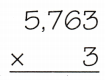Estimated product is 17,400,
Actual product is 17,289.

Explanation:
The product of 5,763×3 is 17,289 and the estimated product is 5,800×3 which is 17,400.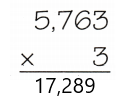Question 16.
Estimate: __________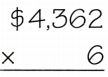Estimated product is 26,400,
Actual product is 26,172.

Explanation:
The product of 4,362×6 is 26,172 and the estimated product is 4,400×6 which is 26,400.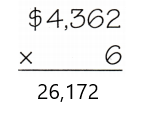Use a strategy to find the product.

Question 17.
3 × 6 × 10 __________
3 × 6 × 10 = 180.

Explanation:
Here, Associative Property states that the product of three or more numbers remains the same regardless of how the numbers are grouped. So
3 × 6 × 10 = (3 × 6) × 10
= 18×10
= 180.

Question 18.
12 × 50 __________
12 × 50 = 600.

Explanation:
Here, Distributive property means if we multiply a sum by a number is the same as multiplying each addend by the number and adding the products. So
12 × 50 = (6+6)× 50
= (6× 50)+(6× 50)
= 300+300
= 600.

Question 19.
5 × 15 __________
5 × 15 = 75.

Explanation:
Here, Distributive property means if we multiply a sum by a number is the same as multiplying each addend by the number and adding the products. So
5 × 15 = 5 ×(10+5)
= (5 × 10)+(5 × 5)
= 50+25
= 75.

Question 20.
8 × 120 __________
8 × 120 = 960.

Explanation:
Here, Distributive property means if we multiply a sum by a number is the same as multiplying each addend by the number and adding the products. So
8 × 120 = 8 ×(100+20)
= (8 × 100)+(8 × 20)
= 800+160
= 960.

Question 21.
Sunset School had 4 performances of their school play during the first week. The auditorium can seat 2,518 people and all of the seats at each performance were filled. During the second week, 7,450 people attended the play. How many people saw the play during the two weeks?
(A) 10,072
(B) 4,932
(C) 9,968
(D) 17,522
C.

Explanation:
Given that the auditorium can seat 2,518 people and all of the seats at each performance were filled for first week and during the second week, 7,450 people attended the play, so the number of people saw the play during the two weeks is 2,518+7,450 which is 9,968 people.

Question 22.
The table below shows the number of calories in 1 cup of different kinds of berries. How many more calories are in 4 cups of blackberries than in 4 cups of strawberries?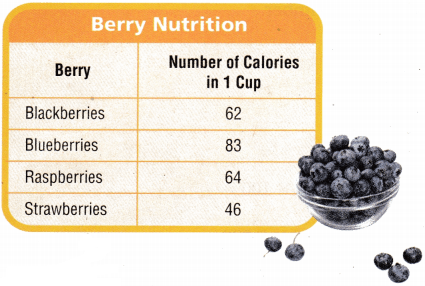(A) 16
(B) 184
(C) 64
(D) 248
C.

Explanation:
Given that 1 cup of blackberries has 62 calories, so for 4 cups it will be 62×4 which is 248 calories. And 1 cup of strawberries has 46 calories, so for 4 cups it will be 46×4 which is 184 calories. So the number of more calories are in 4 cups of blackberries than in 4 cups of strawberries is 248-184 which is 64 calories.

Question 23.
The skating rink rents 184 pairs of skates in a month. About how many pairs of skates does the rink rent in 4 months?

Record your answer and fill in the bubbles on the grid. Be sure to use the correct place value.